Kusudama Star
 What ist the Kusudama Star? Folding the Kusudama Module Assembling the star My Experiences with Notes Some Mathematics Kusudama Stars on the Internet.
 To the Main Page    "Mathematische Basteleien"

What ist the Kusudama Star?
 ...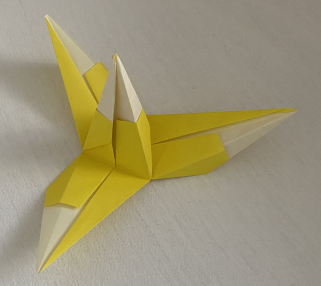... This is the first of 20 pyramids On the internet I found under the name Kusudama Star a series of stars which, like the Bascetta and Sonobe stars, are folded of 30 modules and which are also stellated icosahedra.  For this page I have chosen the "Origami Igel Kusudama", whose construction is shown in a Youtube video (belongs to "Katrin Origami Page"). The inventor of the module and the star is Ekaterina Lukasheva.
I have chosen the star because the pyramids are more pointed and thus more handsome than the pyramids of the Bascetta star. - It is remarkable, that the module uses the creation of a 30° angle by folding alone (step 4 below).

There are own pages for the Sonobe Star and the Bascetta Star.
 .... Bascetta Star..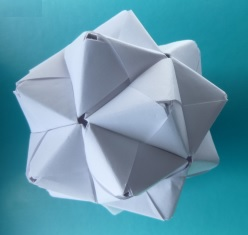Sonobe Star

Folding the Kusudama Module     top
Materials
 ...... You need 30 squares of paper for the star. The 9cm x 9cm notepads that you can buy in stationery shops would be a good choice. They were good for the Bascetta and the Sonobe Star. But for this star they are unsuitable in my opinion. You have to use thin origami paper like shown in the video. I did that and it worked better.

12 Steps
1
 ...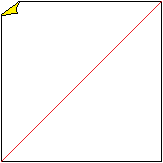.... Fold on the red line and unfold again.2
 ............ Fold on the red line and unfold again.3
 ...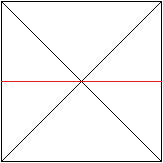... Fold on the red line and unfold again.4
 ...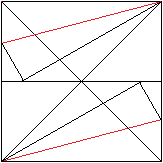... Fold on the red lines so that the corners of the square touch the centre line.5
 ...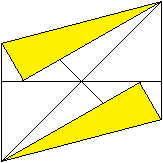... This should look like the picture on the left.6
 ...... Fold on the red lines. The lower line and the upper line should meet the diagonal.7
 ...It should then look like this.8
 ...Turn over the module and fold on the red lines. Unfold again.9
 ...Fold on the red line.10
 .... It should then look like this. Unfold.11
 ...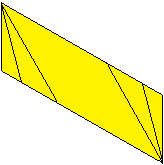It should then look like this.12
 ...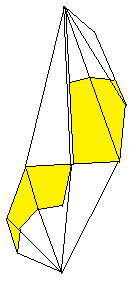Turn the module over, place the tips on top of each other, fold and unfold.  This is the module.Assembling the Star top
 ...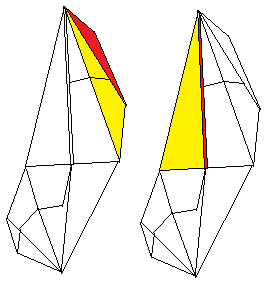Connect two modules. To do this, put the red flap into the red pocket. Then the yellow triangles lie on top of each other....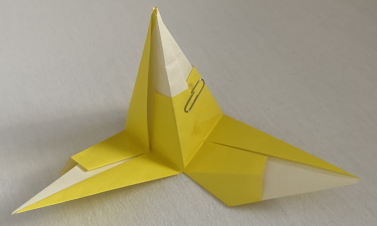... It should look like this. The flap did not stick in the pocket; a paper clip helps........... Enclose the two connected modules with a third module. The first pyramid is finished.  Then add more modules step by step and form more pyramids. When doing this make sure that five pyramids always form a wreath....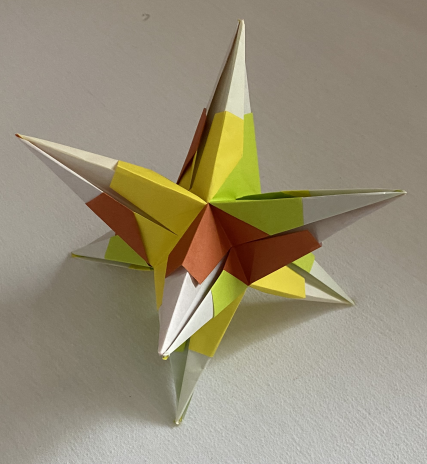... At this point, the finished Kusudama star with 20 pyramids should actually be stand.  For various reasons, I offer a smaller star made of 12 modules with eight pyramids. The star is a stellated octahedron in which a wreath always consist of four pyramids.

My Experiences with Notes  top
 ...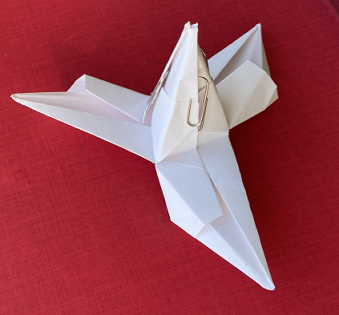... ...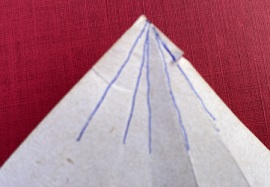... If the modules are folded from notepads, the result is unsatisfactory.   - As you can see on the left, the tip of the pyramid is frayed. It is hardly possible to make five folding lines at one corner of the notepads. The paper also often tears.   - Then the pockets are so open and the flaps so small that I need paper clips to hold the modules together.

Some Mathematics top
 ...... If you unfold the module, you will recognise the side faces of two pyramids as isosceles triangles.  The angle at the top  of a triangle is 30° because of the folding process, the height of a triangle is half the diagonal of the square with the side a.

Maybe three questions are interesting.
1st Question
Only a small part of the square area Aq of the module is used. What percentage is this?

 ...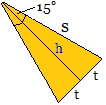... Ahead: tan(15°) = 2-sqrt(3) In the half isosceles triangle the height h=(1/2)sqrt(2)*a and the angle of 15° are known.  tan(15°) = t/h then t = h/tan(15°) = [sqrt(2)-(1/2)sqrt(6)]a = 2*[2sqrt(2)-sqrt(6)]a . The area of the side face of a pyramid is A = (1/2)(2t)h = th = (1/2)[2-sqrt(3)]a² or rounded 0,134a².
Two isosceles triangles contribute to the formation of the star. They have the area 2A,
The square of the module has the area Aq = a², then rounded 2A/Aq = 0,268.
Result
About 27% of the area of the initial square is used.

2nd Question
What is the height H of a pyramid?

Antwort
 ...... Ahead: cos(15) = (1/4)[sqrt(2)+sqrt(6)] s = h/cos(15°) =[2sqrt(2)/(sqrt(2)+sqrt(6))]a = [sqrt(3)-1]a. The height in the equilateral triangle with side 2t is h' = (1/2)sqrt(3)(2t).

 ...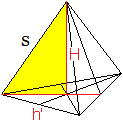... According to the theorem of Pythagoras the following applies  H² = s²-[(2/3)h']² = [sqrt(3)-1]²a²-[(2/3)(1/2)sqrt(3)2t]a² = [sqrt(3)-1]²a²-[(2/3)sqrt(3)t]a² =[sqrt(3)-1]²a²-[(2/3)sqrt(3)(sqrt(2)-(1/2)sqrt(6))]²a² = [sqrt(3)-1]²a²-[2/3sqrt(6)-sqrt(2)]²a² = [4-2sqrt(3)]²a²-[14/3-8/3sqrt(3)]a² = [-2/3+2/3sqrt(3)]a². Then H = (1/3)sqrt[6sqrt(3)-6]a.
Result
The height of a pyramid is H = (1/3)sqrt[6sqrt(3)-6]a or rounded H = 0.699a.
If the side length of the initial square is a = 9 cm, the height is H = 6.3 cm.

3rd Question
How big is the star?
This means the distance e of the tops of two opposite pyramids.

The distance between opposite side faces of the icosahedron is twice the radius of the incircle.
d = (1/6)[3sqrt(3)+sqrt(15)](2t) = (1/6)[3sqrt(3)+sqrt(15)][2sqrt(2)-sqrt(6)]a or rounded d=0.571a.
The distance is e = 2H+d = 2*0.699a+0.573a = 1.971a.
Result
If the side length of the initial square is a = 9.0 cm, the size of the star will be 17.7 cm.

Comparison of three stars
 a=9,0cm Sonobe Star Bascetta Star Kusudama Star 2A/Aq 12,7% 20,0% 26,8% H 0,29a = 2,6cm 0,49a = 4,4cm 0,70a = 6,3cm e 1,04a = 9,4 cm 1,45a = 13,1cm 1,97a = 17,7cm

 Sonobe Star Bascetta Star Kusudama Star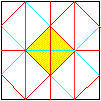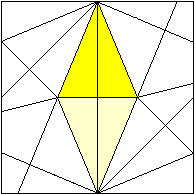Kusudama Stars on the Internet    top

Ten Links to Stars with 20 Pyramids

1.Stern: Sonobe-Stern

2.Stern: Bascetta-Stern

3. Stern: Origami Igel Kusudama (Youtube)

Und weiter

5. Stern: Origami Modular Star  (Bascetta-Stern, gespiegelt)

6. Stern: How to fold an Origami Modular Star (Youtube)

7. Stern: 30-unit Sky Star Kusudama (Creator: Maria Sinayskaya) (Youtube)

8. Stern: Origami Kusudama/ 3D Star Ball for Christmas/ Paper 30-point Star (Youtube)

9. Stern: Origami Estramallar Origami (Youtube)

10. Stern: Tutorial for Origami Sonobe Variation Kusudama 30 (Youtube)

Elisa (japanliebe.de)
kusudama  was haben origami-Bälle mit Medizin zu tun?

Wikipedia
Kusudama

PopUp Star

Kusudama estrella Ostrum